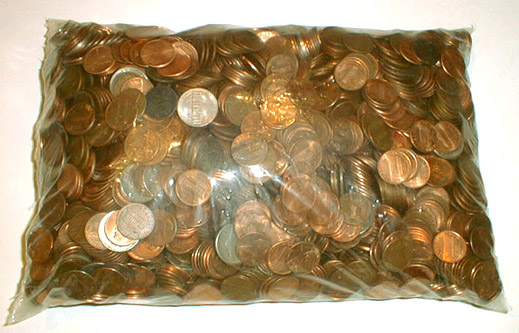to english | index | next

2620枚の硬貨から成る29米ドル24セント (金額第二八番)
US\$29.24 Which Consists of 2620 Coins (Money Amount No. 28)

The U. S. coins, a plastic bag
2002

cf. 2620 and 2924 are the third smallest amicable pair which Euler found in 1747.
(Two numbers are called amicable if each equals to the sum of the divisors of the other.)
Divisors of 2620: 1 + 2 + 4 + 5 + 10 + 20 + 131 + 262 + 524 + 655 + 1310 = 2924
Divisors of 2924: 1 + 2 + 4 + 17 + 34 + 43 + 68 + 86 + 172 + 731 + 1462 = 2620